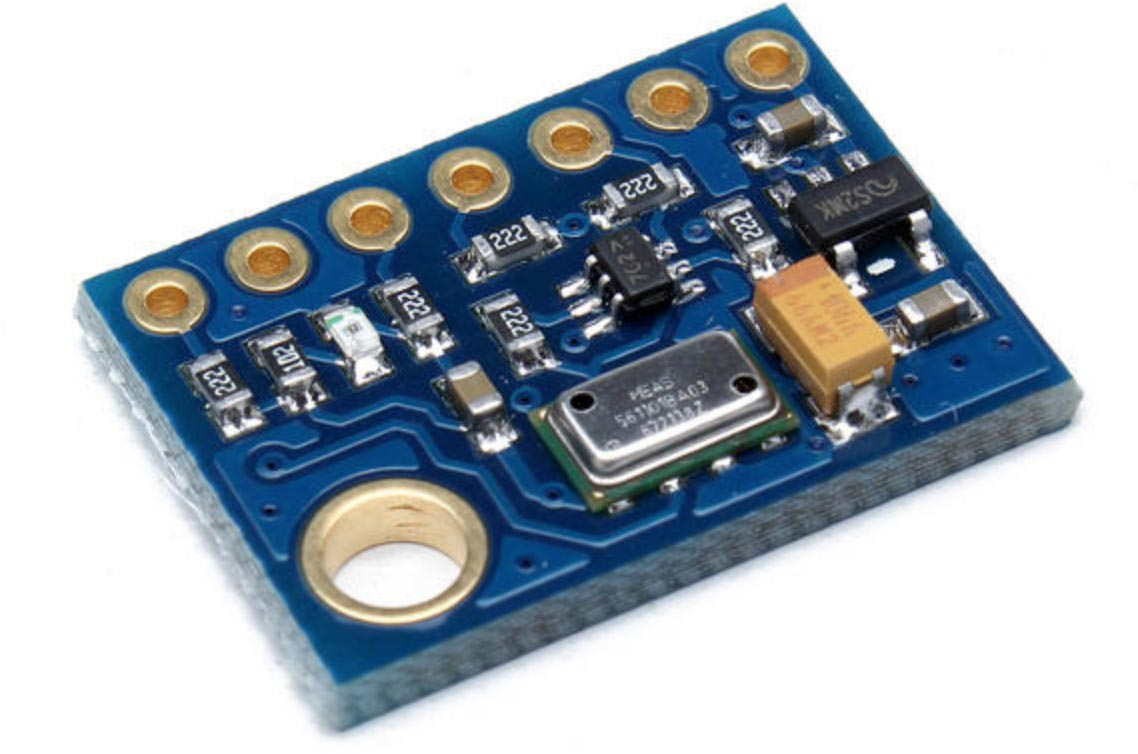## MS5611 Pressure Sensor

The MS5611  is a high resolution altimeter sensor from MEAS Switzerland with SPI and I2C bus interfaces. It has an operating voltage range of 1.8 V and 3.6 V, so you'll need a regulator and logic-level conversion if you want to use it on a 5 V board.

Breakout boards are available from sites such as eBay and Banggood :To use the I2C interface take the PS pin high. The CSB pin can be used to choose between two I2C addresses; the default I2C address with CSB connected to GND is #x77 or 119. These are the default settings on the breakout board if you leave PS and CSB unconnected.

This sensor requires floating-point calculations, so it's only suitable for 32-bit versions of uLisp. The following routines use the I2C interface.

#### Initialising the sensor

The routine readprom reads the configuration values C1 to C6 from the PROM into the variable config. You should call this once before using the sensor:

```(defvar config nil)

(let (c)
(dotimes (n 6)
(with-i2c (str #x77)
(write-byte (+ #xA2 (* 2 n)) str)
(restart-i2c str 2)
(setq config (reverse c))))
```

#### Reading the temperature and pressure

```(defun adc ()
(delay 10)
(with-i2c (str #x77)
(write-byte #x00 str)
(restart-i2c str 3)

(let ((c (lambda (x) (nth (1- x) config)))
d1 d2)
; Convert D1 with OSR = 4096
(with-i2c (str #x77)
(write-byte #x48 str))
; Convert D2 with OSR = 4096
(with-i2c (str #x77)
(write-byte #x58 str))
; calculate the temperature and pressure as in datasheet
(let* ((dt (- d2 (* (c 5) 256)))
(temp (/ (+ 2000 (* dt (/ (c 6) 8388608))) 100))
(off (+ (* (c 2) 65536) (/ (* (c 4) dt) 128)))
(sens (+ (* (c 1) 32768) (/ (* (c 3) dt) 256)))
(p (/ (/ (- (/ (* d1 sens) 2097152) off) 32768) 100)))
(list temp p))))```

The temperature and pressure are returned as a list of two values, temperature in °C and pressure in hPa; for example:

```> (read)
(23.1468 985.334)```

Thanks to Ronny Suy for the routines on which these were based.

1. ^ MS5611 Datasheet on TE Connectivity.
2. ^ MS5611 GY-63 Atmospheric Pressure Sensor Module on Banggood.